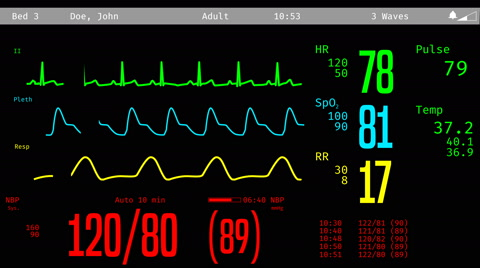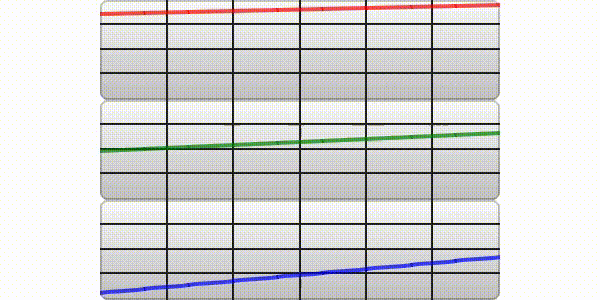# Multi Chart in Single Window using LittleVGLHi,

I’m currently working on a Patient Monitoring Project. I need to know whether I can make this kind of multi-chart in a single window. I’ve done a single chart in a window using LittleVGL but can we do multi-chart using LittleVGL??.

You can create multiple chart objects and update them all at the same time.

Is there any demo file. I’ve searched but I didn’t found anything related to it.

There is a demo for a single chart object in the chart documentation. Depending on your needs, you can then create multiple series on the chart, or multiple chart objects.

Here is code that I just create for example of multi chart object:

``````/*
* chart.c
*
*  Created on: Jan 6, 2020
*      Author: teguh
*/

#include "chart.h"

static lv_obj_t * chart_one;
static lv_obj_t * chart_two;
static lv_obj_t * chart_three;

static lv_chart_series_t * ser_one;
static lv_chart_series_t * ser_two;
static lv_chart_series_t * ser_three;

static char point_one = 0;
static char point_two = 0;
static char point_three = 0;

void chart_create(void){

/*Create a chart one*/
chart_one = lv_chart_create(lv_scr_act(), NULL);
lv_obj_set_size(chart_one, 400, 100);
lv_obj_align(chart_one, NULL, LV_ALIGN_IN_TOP_MID, 0, 0);
lv_chart_set_type(chart_one, LV_CHART_TYPE_LINE);
lv_chart_set_series_opa(chart_one, LV_OPA_70);
lv_chart_set_series_width(chart_one, 4);
lv_chart_set_range(chart_one, 0, 100);

/*Create a chart two*/
chart_two = lv_chart_create(lv_scr_act(), NULL);
lv_obj_set_size(chart_two, 400, 100);
lv_obj_align(chart_two, NULL, LV_ALIGN_CENTER, 0, 0);
lv_chart_set_type(chart_two, LV_CHART_TYPE_LINE);
lv_chart_set_series_opa(chart_two, LV_OPA_70);
lv_chart_set_series_width(chart_two, 4);
lv_chart_set_range(chart_two, 0, 100);

/*Create a chart three*/
chart_three = lv_chart_create(lv_scr_act(), NULL);
lv_obj_set_size(chart_three, 400, 100);
lv_obj_align(chart_three, NULL, LV_ALIGN_IN_BOTTOM_MID, 0, 0);
lv_chart_set_type(chart_three, LV_CHART_TYPE_LINE);
lv_chart_set_series_opa(chart_three, LV_OPA_70);
lv_chart_set_series_width(chart_three, 4);
lv_chart_set_range(chart_three, 0, 100);

/*Create a series three*/
ser_one = lv_chart_add_series(chart_one, LV_COLOR_RED);      /*Create a series one*/
ser_two = lv_chart_add_series(chart_two, LV_COLOR_GREEN);    /*Create a series two*/
ser_three = lv_chart_add_series(chart_three, LV_COLOR_BLUE); /*Create a series three*/

/*Create task for filling the Charts*/
}

{
(void) param;    /*Unused*/

/*Summing point one*/
point_one = point_one + 1;
if(point_one > 100){
point_one = 0;
}

/*Summing point two*/
point_two = point_two + 2;
if(point_two > 100){
point_two = 0;
}

/*Summing point three*/
point_three = point_three + 4;
if(point_three > 100){
point_three = 0;
}

lv_chart_set_next(chart_one, ser_one, point_one);       /*set point_one to next*/
lv_chart_set_next(chart_two, ser_two, point_two);       /*set point_two to next*/
lv_chart_set_next(chart_three, ser_three, point_three); /*set point_three to next*/

}
``````I hope the comment code is easily understandable for your reference.

Regards,
TJ.

2 Likes

Thanks! By the way, you can get rid of the backgrounds with `lv_chart_set_style(chart, LV_CHART_STYLE_MAIN, &lv_style_transp)`.

2 Likes

It worked perfectly. Thanks for the immediate support.